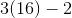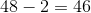Example Questions

Example Question #711 : Algebra

Simplify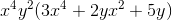.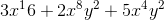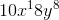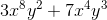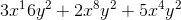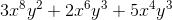Explanation:

This is just a matter of simply distributing this multiplication. Start by the basic distribution: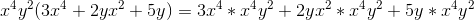Now, you just add the exponents that are similar. Thus, you get:Example Question #712 : Algebra

Simplify the following: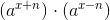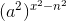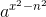None of these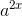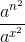Explanation:

The rule for multiplying exponents is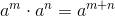.

Using this, we see that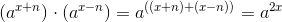.

Thus, our answer is.

Example Question #21 : Exponential Operations

The expression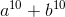is equivalent to which of the following?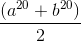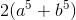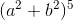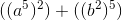None of theseExplanation:

The formula for multiplying exponents is.

Using this, we see that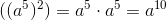, and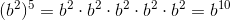.

Example Question #22 : Exponential Operations

Simplify the following: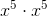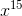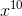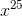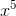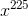Explanation:

When two variables with exponents are multiplied, you can simplify the expression by adding the exponents together. In this particular problem, the correct answer is found by adding the exponents 5 and 5, yielding.

Example Question #23 : Exponential Operations

Simplify the following to its simplest exponential expression: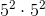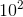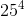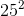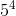Explanation:

When multiplying exponential expressions, the bases remain the same and the exponents are added. Thus, the answer to this question is.

Example Question #24 : Exponential Operations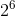can be written as which of the following?

A.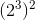B.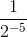C.A only

A, B and C

B and C

A and C

C only

A and C

Explanation:

B is not equivalent because...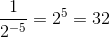A is equivalent because of a property of exponents meaning that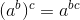. Consequently,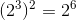C is simply computing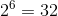.

Example Question #25 : Exponential Operations

Solve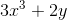whenand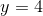.Explanation:

Substituteforandfor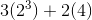Simplify: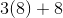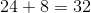Example Question #26 : Exponential Operations

What is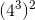?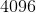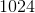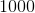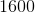Explanation:

When an exponent is raised to an exponent, you may simplify by multiplying the exponents together to make a new exponent. In this case,becomes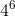, which equals.

Example Question #27 : Exponential Operations

Simplify: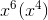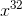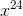Explanation:

When exponents with the same base are being multiplied, you may add the exponents together to create a new exponent.

In this case, you would add 6 and 4 to create 10 as the new exponent.

Keeping the same base, the answer becomes.

Example Question #28 : Exponential Operations

Solve: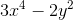whenand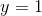.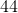Substituteforandfor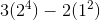.
Simplify: